Custom SearchCurrent flows through the tube for one-half cycle. Current flows through the tube for less than one-half cycle. Current flows through the tube for the entire cycle. MU AND TRANSCONDUCTANCE In your study of triodes so far, you have seen that the output of a triode circuit is developed across the tube. The output is caused by the voltage dropped across RL due to current flow from tube conduction. In all the demonstrations of gain, we assumed that RL was held constant and current through the tube was varied. In this manner we achieved a voltage gain. If the resistance of RL is changed by the designer, the gain of a triode circuit can be either increased or decreased. This is fairly easy to understand. Assume that a circuit is composed of a triode with a plate-load resistor of 100 kohms. If a +2 volt signal causes 2 additional milliamperes to conduct through the tube, the voltage drop across RL (the output) will be: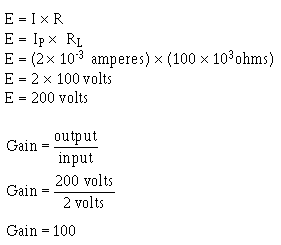Thus, the gain of the circuit is 100. If the plate-load resistor is reduced to 50 kohms and the input is kept at +2 volts, the gain will be reduced to: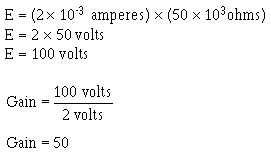As you can see, voltage gain depends on both the tube characteristics and the external circuit design. The voltage gain is a measure of circuit efficiency, not tube efficiency. The actual characteristics of a tube are measured by two factors: mu(μ) or AMPLIFICATION FACTOR; and TRANSCONDUCTANCE or gm. The amplification factor (represented as μ) of a tube is equal to the ratio of a change in plate voltage to the change in grid voltage required to cause the same change in plate current. This is expressed mathematically as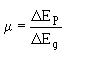While this may sound complicated, it really isn't. Look at figure 1-28. Here you see in view A a triode with a +1 volt input signal. At this grid voltage, current through the tube is at 1 milliampere. If the input voltage is raised to +3 volts, current through the tube increases to 2 milliamperes. The change in Eg (ΔE g) is then 2 volts. This is shown in view B. Suppose that the grid voltage is returned to +1 volt, and the plate voltage is increased until the ammeter in view C reads 2 milliamperes of plate current. At this point plate voltage is measured. Plate voltage had to be increased by 100 volts (350 - 250) to get the same change in plate current (1 mA). The change in plate voltages (ΔEp) is then 100 volts. The amplification factor (μ) of just the tube is then equal to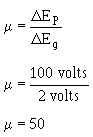Figure 1-28. - Obtaining gain and transconductance.As you can see, mu is a measure of the ability of a tube to amplify. By comparing the mu of two different types of tubes, you can get an idea of their efficiency. For example, assume you have two different tubes, one with a mu of 50, and the other with a mu of 100. If you place each tube in a circuit whose input varies by 2 volts, you can expect the following changes in plate voltage. Tube 1:Tube 2: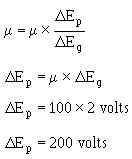Thus, you can expect twice the change in plate voltage from tube 2 as from tube 1 for the same input voltage. Therefore, tube 2 will have twice the gain of tube 1. Transconductance Transconductance is a measure of the change in plate current to a change in grid voltage, with plate voltage held constant. The unit for conductance is the mho (siemens), pronounced "moe." Transconductance is normally expressed in either micromhos or millimhos. Mathematically, transconductance is expressed by the formula: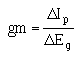Examine figure 1-28, views A and B, again. In view A, the input voltage is +1 volt. At +1 volt Eg, the plate current is equal to 1 milliampere, with a plate voltage of 250 volts. In view B, the input voltage (Eg) is raised to +3 volts. ΔE g, as before, is equal to 2 volts. This increase in grid voltage causes plate current to increase to 2 milliamperes. The change in plate current (ΔIp) is then equal to 1 milliampere. Thus, transconductance (gm) is equal to:Remember that the voltage gain of a circuit is measured by the ratio of the change in plate voltage to the change in grid voltage. Because plate voltage is developed across a resistor, the more current varies with a given input signal, the greater will be the output (E = I X R). If you have two tubes, one with a gm of 500 mhos and the other with a gm of 500 μmhos, you can estimate the relative gain of these two tubes. Assume that the circuit in which you wish to use one of these tubes has a load resistor of 100 kohms and that ΔEg will be 2 volts. The voltage gain of these two circuits will be: Tube 1: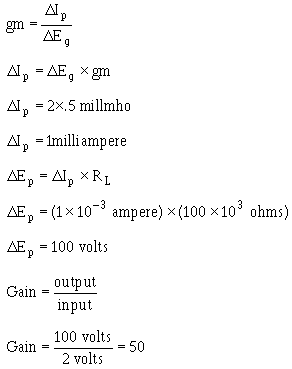Tube 2: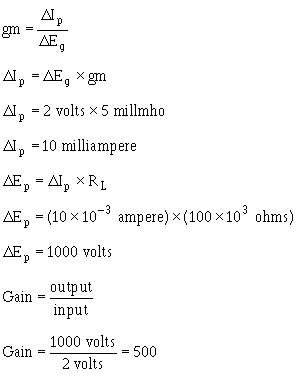As you can see, tube 2 is 10 times the amplifier that tube 1 is. Q.26 The plate voltage of a tube will vary 126 volts when a 3-volt ac signal is applied to the control grid. What is the gain of this tube?Q.27 If the mu of a tube is 85 and the signal at the control grid is 4 volts ac, the plate voltage will vary by what amount?Q.28 Transconductance is a measure of the relationship between what two factors?Q.29 A tube has a transconductance of 800 mhos and a load resistor of 50 kohms. When control grid voltage varies by 6 volts, the plate voltage will vary by what amount?